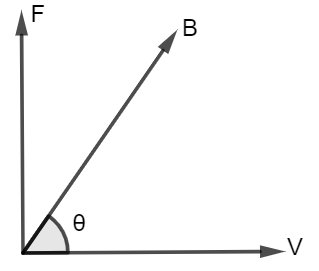Question

# A charge of +10 C enters a uniform magnetic field parallel to its direction. What will happen to the charge?

Open in App
Solution

## Magnetic field:A magnetic field is a region around the magnet.The magnetic field which moving the electric charge.It possesses the force of magnetism acts.A diagram to represent the magnetic field:Explanation:As we know that a charged particle's magnetic force is orthogonal to the magnetic field.So, $F=qvB\mathrm{sin}\theta$So, in a straight line, there is no net force, and the charged particle goes if its velocity is parallel to the magnetic field.Therefore, the angle between the magnetic field vector B and the velocity vector forms the force.And, the magnetic force is equal to $F=qvB\mathrm{sin}\theta$So, if the velocity and magnetic field are parallel and no force occurs, then in this condition a charged particle can continue to move in a straight line.Suggest Corrections0Similar questions